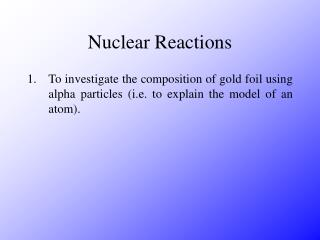DownloadDownload PresentationNuclear Reactions

# Nuclear Reactions

Télécharger la présentation## Nuclear Reactions

- - - - - - - - - - - - - - - - - - - - - - - - - - - E N D - - - - - - - - - - - - - - - - - - - - - - - - - - -
##### Presentation Transcript

1. Nuclear Reactions • To investigate the composition of gold foil using alpha particles (i.e. to explain the model of an atom).

2. vacuum q a-source zinc sulphide detector gold foil Nuclear Reactions • The equipment used:

3. Nuclear Reactions • Rutherford fired alpha particles through a piece of gold foil and used a zinc sulphide detector to detect the scattered alpha particles and their location. • Alpha particles were used as they would not become attracted to the nucleus.

4. Nuclear Reactions • Rutherford found that 1. Most of the alpha particles passed through the gold foil undeviated, 2. A few alpha particles were deviated from their path but continued through the gold foil, and 3. A small number of alpha particles rebounded. • Rutherford concluded that 1. most of the atom was empty space, 2. The alpha particles were deviated from their original path due to positive charges within the atom, and 3. The atom had a positive nucleus where most of the mass was concentrated.

5. Nuclear Reactions • Rutherford’s model of the atom: He called the heavy positively charged centre the nucleus. He went on to suggest that the nucleus was surrounded by orbiting electrons required for electrical neutrality.

6. Mass number = number of protons + number of neutrons Atomic number = number of protons Nuclear Reactions • Symbols as follows: A X Element symbol Z

7. Nuclear Reactions • Subtract the atomic number from the mass number – A – Z. • An isotope of any particular element contains the same number of protons, but different number of neutrons.

8. 235 238 U U 92 92 Nuclear Reactions • Complete the following tables:

9. Nuclear Reactions • Radioactive decay occurs when unstable atoms become stable by emitting particles and energy. • Energy is always produced as a result of radioactive decay. • The three types of decay that exist are alpha, beta and gamma. • Alpha particles are equivalent to a Helium nucleus.

10. A A - 4 X Y + Z Z - 2 4 He 2 Nuclear Reactions • The alpha decay equation rule: • A beta particle is equivalent to a fast moving electron.

11. A A 0 b X Y + Z Z + 1 -1 Nuclear Reactions • Beta decay occurs when a neutron changes into a proton and an electron. The electron is ejected from the atom at high speed. As a result, the mass number remains the same whilst the atomic number increases by 1. • The beta decay equation rule:

12. Nuclear Reactions • When atoms decay by emitting a or b particles to form a new atom, the nuclei of the new atom formed may still have too much energy to be completely stable. The excess energy is emitted as a gamma ray which is a form of electromagnetic radiation.

13. Nuclear Reactions • Nuclear fission is the process whereby a nucleus, with a high mass number, splits into 2 nuclei which have roughly equal mass numbers. • The 2 types of nuclear fission that exist are spontaneous fission and induced fission. • Neutrons and energy are always released as a result of nuclear fission. • Nuclear fission can be induced by bombarding an atom with neutrons.

14. Nuclear Reactions • The kinetic energy before nuclear fission takes place is a lot less than the kinetic energy after nuclear fission takes place. • Nuclear fusion is the process whereby 2 nuclei, with low mass numbers, combine to produce a single nucleus with a higher mass number. • Neutrons and energy are released as a result of nuclear fusion.

15. 222 218 4 4 Rn Po He He + 86 84 2 2 Nuclear Reactions • E = mc2 • E = 2.385 x 10-11 J • The following equation:

16. 0 b -1 234 234 Th Pa + 90 91 Nuclear Reactions • The following equation: • Nuclear fission. • Beta decay. • Nuclear fusion. • Alpha decay.